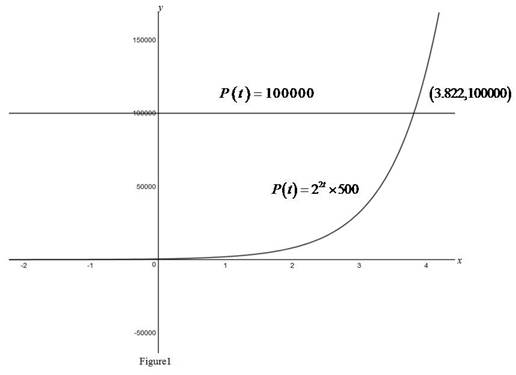# The population of bacteria after 3 hours.### Single Variable Calculus: Concepts...

4th Edition
James Stewart
Publisher: Cengage Learning
ISBN: 9781337687805### Single Variable Calculus: Concepts...

4th Edition
James Stewart
Publisher: Cengage Learning
ISBN: 9781337687805

#### Solutions

Chapter 1.5, Problem 30E

(a)

To determine

## To Find: The population of bacteria after 3 hours.

Expert Solution

There are 32,000 bacteria after 3 hours.

### Explanation of Solution

Given:

The initial population of bacteria is 500 and it gets double for every half hour.

Calculation:

Using the given initial population of bacteria P(0) and the rate of growth, define a function to calculate the population of bacteria in terms of time t as shown below,

P(t)=2t0.5P(0)=22tP(0)=4tP(0)

Therefore, P(t)=4t×P(0)

Substitute t=3 to get the population of bacteria after 3 hours.

P(3)=22(3)×500=26×500=64×500=32,000

Therefore, there are 32,000 bacteria after 3 hours.

(b)

To determine

### To find: The population of bacteria after t hours.

Expert Solution

There are 4t×500 bacteria after t hours.

### Explanation of Solution

Given:

The initial population of bacteria is 500 and it gets double for every half hour.

Calculation:

Using the given initial population of bacteria P(0) and the rate of growth, define a function to calculate the population of bacteria in terms of time t as shown below,

P(t)=2t0.5P(0)=22tP(0)=4tP(0)

Therefore, P(t)=4t×P(0)

Substitute P(0)=500 and obtain the expression for population of bacteria after t hours.

Therefore, there are 4t×500 bacteria after t hours.

(c)

To determine

### To Find: The population of bacteria after 40 minutes.

Expert Solution

There are 1260 bacteria after 40 minutes.

### Explanation of Solution

Given:

The initial population of bacteria is 500 and it gets double for every half hour.

Calculation:

Using the given initial population of bacteria P(0) and the rate of growth, define a function to calculate the population of bacteria in terms of time t as shown below,

P(t)=2t0.5P(0)=22tP(0)=4tP(0)

Therefore, P(t)=4t×P(0)

Convert the given time into hour as shown below,

40minutes = 4060 hours= 23 hours.

Substitute t=23 to calculate the population of bacteria after 23 hours follows,

P(23)=4(23)×500=1613×500=2.52×5001260

Therefore,. there are 1260 bacteria after 40 minutes.

(d)

To determine

Expert Solution

### Explanation of Solution

Graph:

Use online graph calculator and draw the graph of the functions P(t)=4t×500 as shown in the below Figure1.From Figure 1, the curve intersects the line y=100000 at the point (3.822,100000) and that means the time is 3.822 hours when the population becomes 100000.

That is, t=3.822 when P(t)=100000 .

Therefore it takes 3.822 hours to reach to 100000 to the population.

### Have a homework question?

Subscribe to bartleby learn! Ask subject matter experts 30 homework questions each month. Plus, you’ll have access to millions of step-by-step textbook answers!